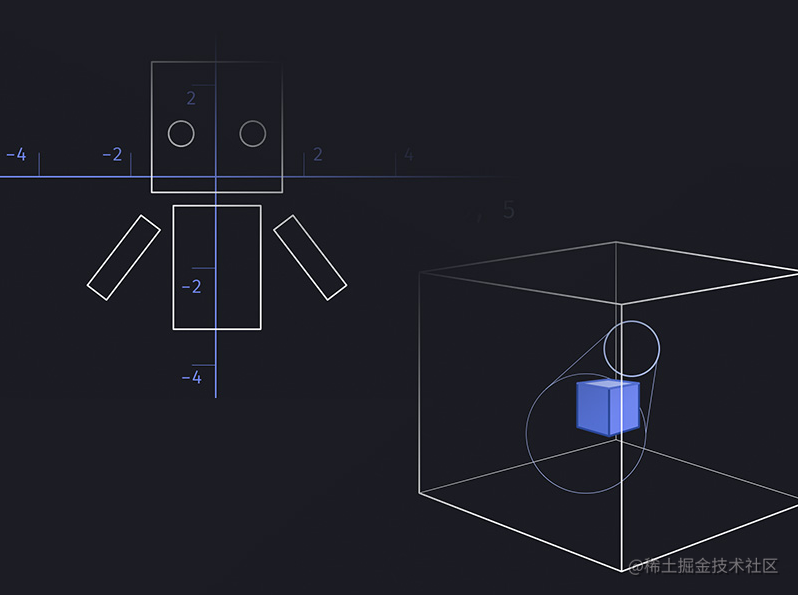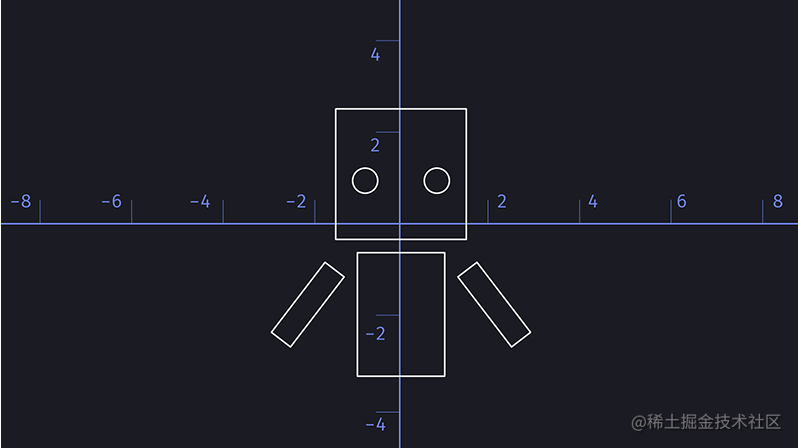# [译文]在 Three.js 中创建 3D 角色Three.js是一个 JavaScript 库，用于使用 WebGL 进行 3D 绘图。它使我们能够将 3D 对象添加到场景中，并操纵位置和照明等内容。如果您是一个习惯于使用 CSS 处理 DOM 和样式元素的开发人员，那么 Three.js 和 WebGL 看起来像是一个全新的世界，而且可能有点吓人！本文适用于熟悉 JavaScript 但对 Three.js 相对较新的开发人员。我们的目标是通过 Three.js（一个 3D 动画人物）构建一些简单但有效的东西，以掌握基本原理，并证明一点知识可以带您走很长的路！

## 设置场景

### 基本原则

``<canvas data-canvas></canvas>``

``````const canvas = document.querySelector('[data-canvas]')

// Create the scene
const scene = new THREE.Scene()

// Create the camera
const camera = new THREE.PerspectiveCamera(75, sizes.width / sizes.height, 0.1, 1000)

// Create the renderer
const renderer = new THREE.WebGLRenderer({ canvas })

// Render the scene
renderer.setSize(window.innerWidth, window.innerHeight)
renderer.render(scene, camera)``````

``camera.position.z = 5``

## 3D 形状

Three.js 中的对象称为网格。为了创建网格，我们需要两件事：几何材质。几何图形是 3D 形状。Three.js 有一系列可供选择的几何图形，可以以不同的方式进行操作。出于本教程的目的——看看我们可以用一些基本原理制作哪些有趣的场景——我们将把自己限制在两种几何形状：立方体和球体。

``````// Create a new BoxGeometry with dimensions 1 x 1 x 1
const geometry = new THREE.BoxGeometry(1, 1, 1)``````

``````// Create a new material with a white color
const material = new THREE.MeshLambertMaterial({ color: 0xffffff })``````

``````const mesh = new THREE.Mesh(geometry, material)

``````const lightDirectional = new THREE.DirectionalLight(0xffffff, 1)

``````const lightDirectional = new THREE.DirectionalLight(0xffffff, 1)

// Move the light source towards us and off-center
lightDirectional.position.x = 5
lightDirectional.position.y = 5
lightDirectional.position.z = 5``````

![5, 5, 5 处的灯位]()

``lightDirectional.position.set(5, 5, 5)``

``````const degreesToRadians = (degrees) => {
return degrees * (Math.PI / 180)
}

``````const lightAmbient = new THREE.AmbientLight(0x9eaeff, 0.2)

## 创建一个类

``````class Figure {
constructor(params) {
this.params = {
x: 0,
y: 0,
z: 0,
ry: 0,
...params
}
}
}``````

### 团体

``````class Figure {
constructor(params) {
this.params = {
x: 0,
y: 0,
z: 0,
ry: 0,
...params
}

this.group = new THREE.Group()
}
}``````

## 创建身体部位

``````const material = new THREE.MeshLambertMaterial({ color: 0xffffff })

class Figure {
constructor(params) {
this.params = {
x: 0,
y: 0,
z: 0,
ry: 0,
...params
}

this.group = new THREE.Group()
}

createBody() {
const geometry = new THREE.BoxGeometry(1, 1.5, 1)
const body = new THREE.Mesh(geometry, material)
}
}``````

``````createBody() {
const geometry = new THREE.BoxGeometry(1, 1.5, 1)
const body = new THREE.Mesh(geometry, material)
}

init() {
this.createBody()
}``````

## 将人物添加到场景中

``````const figure = new Figure()
figure.init()``````

``````createHead() {
const geometry = new THREE.BoxGeometry(1.4, 1.4, 1.4)
const head = new THREE.Mesh(geometry, material)

// Position it above the body
}

init() {
this.createBody()
}``````

## 添加手臂

``````createArms() {
for(let i = 0; i < 2; i++) {
const geometry = new THREE.BoxGeometry(0.25, 1, 0.25)
const arm = new THREE.Mesh(geometry, material)

}
}``````

``````init() {
this.createBody()
this.createArms()
}``````

``````createArms() {
for(let i = 0; i < 2; i++) {
const geometry = new THREE.BoxGeometry(0.25, 1, 0.25)
const arm = new THREE.Mesh(geometry, material)
const m = i % 2 === 0 ? 1 : -1

arm.position.x = m * 0.8
arm.position.y = 0.1
}
}``````

``arm.rotation.z = degreesToRadians(30 * m)``### 旋转

1. 创建一个新的 Three.js 组。
2. 将组定位在我们人物的“肩膀”（或我们想要旋转的点）。
3. 为手臂创建一个新网格并将其相对于组定位。
4. 旋转组（而不是手臂）。

``````createArms() {
const geometry = new THREE.BoxGeometry(0.25, 1, 0.25)

for(let i = 0; i < 2; i++) {
const arm = new THREE.Mesh(geometry, material)
const m = i % 2 === 0 ? 1 : -1

// Create group for each arm
const armGroup = new THREE.Group()

// Add the arm to the group

// Add the arm group to the figure

// Position the arm group
armGroup.position.x = m * 0.8
armGroup.position.y = 0.1
}
}``````

``````// Inside the `for` loop:
const box = new THREE.BoxHelper(armGroup, 0xffff00)

``````createArms() {
// Set the variable
const height = 1
const geometry = new THREE.BoxGeometry(0.25, height, 0.25)

for(let i = 0; i < 2; i++) {
const armGroup = new THREE.Group()
const arm = new THREE.Mesh(geometry, material)

const m = i % 2 === 0 ? 1 : -1

// Translate the arm (not the group) downwards by half the height
arm.position.y = height * -0.5

armGroup.position.x = m * 0.8
armGroup.position.y = 0.6

// Helper
const box = new THREE.BoxHelper(armGroup, 0xffff00)
}
}``````

``````// In the `for` loop

### 眼睛

``const geometry = new THREE.SphereGeometry(1, 32, 16)``

``````createEyes() {
const eyes = new THREE.Group()
const geometry = new THREE.SphereGeometry(0.15, 12, 8)

// Define the eye material
const material = new THREE.MeshLambertMaterial({ color: 0x44445c })

for(let i = 0; i < 2; i++) {
const eye = new THREE.Mesh(geometry, material)
const m = i % 2 === 0 ? 1 : -1

// Add the eye to the group

// Position the eye
eye.position.x = 0.36 * m
}
}``````

``````createHead() {
// Create a new group for the head

// Create the main cube of the head and add to the group
const geometry = new THREE.BoxGeometry(1.4, 1.4, 1.4)
const headMain = new THREE.Mesh(geometry, material)

this.createEyes()
}``````

``````// in createEyes()

// Move the eyes forwards by half of the head depth - it might be a good idea to create a variable to do this!
eyes.position.z = 0.7``````

### 腿

``````createLegs() {
const legs = new THREE.Group()
const geometry = new THREE.BoxGeometry(0.25, 0.4, 0.25)

for(let i = 0; i < 2; i++) {
const leg = new THREE.Mesh(geometry, material)
const m = i % 2 === 0 ? 1 : -1

leg.position.x = m * 0.22
}

legs.position.y = -1.15

}``````

## 场景定位

``````class Figure {
constructor(params) {
this.params = {
x: 0,
y: 0,
z: 0,
ry: 0,
...params
}

this.group.position.x = this.params.x
this.group.position.y = this.params.y
this.group.position.z = this.params.z
this.group.rotation.y = this.params.ry
}
}``````

``````const figure = new Figure({
x: -4,
y: -2,
})
figure.init()``````

``new THREE.Box3().setFromObject(figure.group).getCenter(figure.group.position).multiplyScalar(-1)``

## 使其具有生成性

``````const random = (min, max, float = false) => {
const val = Math.random() * (max - min) + min

if (float) {
return val
}

return Math.floor(val)
}``````

``````class Figure {
constructor(params) {
this.bodyHue = random(0, 360)
}
}``````

``````class Figure {
constructor(params) {
this.bodyHue = random(0, 360)

this.bodyMaterial = new THREE.MeshLambertMaterial({ color: `hsl(\${this.bodyHue}, 85%, 50%)` })
}
}``````

## 动画

GSAP 更常用于为 DOM 中的元素设置动画。由于在这种情况下我们没有为 DOM 元素设置动画，因此需要一种不同的方法。GSAP 不需要元素来制作动画——它可以为 JavaScript 对象制作动画。正如 GSAP 论坛上的一篇文章所说，GSAP 只是“快速改变数字”。

``````gsap.to(figure.params, {
repeat: -1,
duration: 20
})``````

``````gsap.ticker.add(() => {
// Update the rotation value
figure.group.rotation.y = this.params.ry

// Render the scene
renderer.setSize(window.innerWidth, window.innerHeight)
renderer.render(scene, camera)
})``````

``````// Set the starting position
gsap.set(figure.params, {
y: -1.5
})

// Tween the y axis position and arm rotation
gsap.to(figure.params, {
y: 0,
repeat: -1,
yoyo: true,
duration: 0.5
})``````

``````/* In the Figure class: */
bounce() {
this.group.rotation.y = this.params.ry
this.group.position.y = this.params.y
}

/* Outside of the class */
figure.bounce()

// Render the scene
renderer.setSize(window.innerWidth, window.innerHeight)
renderer.render(scene, camera)
})``````

``````class Figure {
constructor(params) {
this.arms = []
}
}``````

``````createArms() {
const height = 0.85

for(let i = 0; i < 2; i++) {
/* Other code for creating the arms.. */

// Push to the array
this.arms.push(armGroup)
}
}``````

``````bounce() {
// Rotate the figure
this.group.rotation.y = this.params.ry

// Bounce up and down
this.group.position.y = this.params.y

// Move the arms
this.arms.forEach((arm, index) => {
const m = index % 2 === 0 ? 1 : -1
arm.rotation.z = this.params.armRotation * m
})
}``````

## 包起来

Three.js 还有很多很多，但我们已经看到，只需使用基本的构建块就可以开始构建有趣的东西并不需要太多，有时限制会孕育创造力！如果您有兴趣进一步探索，我推荐以下资源。

### 资源